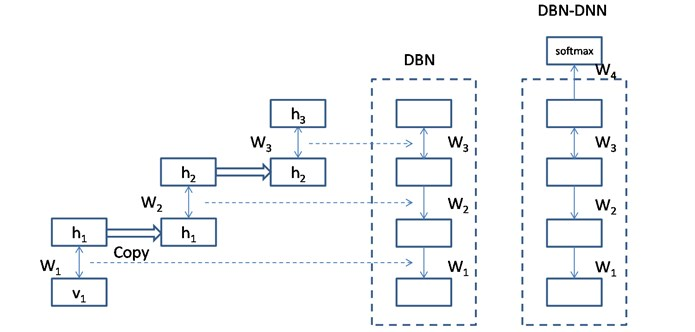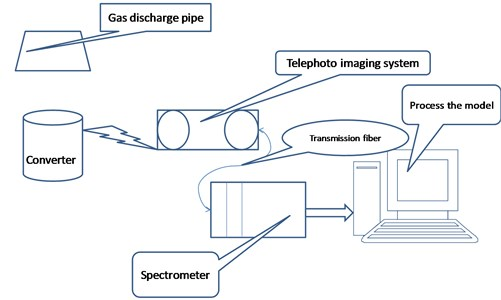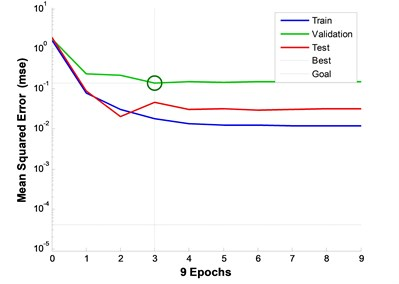Published: 31 March 2018

# The research on intelligent extraction of furnace mouth flame characteristics based on DNN

Lijia Tian1
Jiaying Xing2
Heyu Zhao3
Jincai Chang4
1, 2, 3, 4College of Sciences, North China University of Science and Technology, Tangshan, China
4The Key Laboratory of Data Science and Applications of Hebei Province, Tangshan, China
Corresponding Author:
Jincai Chang
Views 78

#### Abstract

Deep neural networks are a focus of artificial intelligence and big data analysis in recent years. The monitor of the converter mouth is essential to the quality of the steel material production while the requirement of the steel material production is increasingly higher in China. The end-point control of converter blowing is the ultimate regulation of the carbon content and temperature. The severity of carbon-oxygen reaction and the temperature of molten steel can be reflected by the converter mouth flame. Operators judge the end of the steel by watching the converter mouth flame, the converter mouth spark and the time of oxygen supply. So, it is very important to offer a quantitative analysis to converter mouth flame characteristics. We quote the deep neural network into the intelligent extraction of the flame characteristics of the furnace mouth and construct a flame color recognition algorithm based on the deepness letter neural network. This paper belongs to the data science problem in the intelligent research of steel production. By observing the converter flame during the steel flame changes, this paper records the data of light intensity and end-point carbon content of each steel making furnace. When this paper then uses the temperature of flame emission spectrum to deduce and the absorption of the molten steel to judge the contents of the carbon during the converter steel blew process, it is more feasible and accurate than watching by operators. At the same time, by using deep learning algorithm, this paper makes the control process get automatic learning ability and achieve intelligent production so that we can provide a basis for solving the problem of predicting the end-point carbon content in molten steel during the blowing process.

## 1. Introduction

The problem, measurement of carbon content in the online terminal of Converter steel, hasn’t been solved for many years in the metallurgical industry worldwide. At present, some domestic steel enterprises are mainly controlled by a single static model. Because of the lack of dynamic information in the furnace, the model can’t be corrected constantly, so operators cannot get the real-time testing of the furnace reaction conditions . So, this paper uses manual control as a supplement and control the process through the real-time changes in the flame. In this way, the booster blowing rate isn’t increasing significantly and add the labor intensity to operators. U. Jia, come from Beijing University of Science and Technology, uses the texture analysis method in flame image processing technology to analyze and calculate the texture features of flames in each image and judge the ending point according to the change of the texture features of these textures . Ji Xiang, together with some people, purposes that they can analyze the brightness and texture of the flame simultaneously and this provides a new method to solve the problem . They propose a feature extraction method for establishing multi-layer neural network learning on unsupervised data, inspired by the hierarchy of information processing in the human visual perception system, it has stronger unsupervised feature learning ability. It explains the changes in the flame combustion in converter steel. It also analyzes the changes in the characteristics of the flame and what is relevant. From the perspective of data research and data science, this paper uses deep neural network algorithm to analyze the characteristics of gas chromatography of furnace mouth and builds a model of relationship between spectral information and molten steel carbon content to solve the problem of online end point carbon content in steel process accurate prediction of the problem and provide a theoretical basis for converter steel .

## 2. The current development of DNN

In 2006, Geoffrey Hinton and his team published a paper on deep faith network training algorithms, introducing an efficient unsupervised learning method to assist the training of deep structure networks, breaking the long-standing problem the depth of the network is difficult to train . The characteristic of deep learning is automatic learning from big data. Good features can improve the performance of the pattern recognition system. Over the past few decades, hand-designed features have dominated the applications of pattern recognition.

Deep learning can be characterized by automatic learning of big data, which can contain thousands of parameters. It takes five to ten years to manually design effective features, while deep learning can be automatically learned from the training data for new applications to new valid features to represent. A pattern recognition system includes both feature and classifier. In the traditional approach, the optimization of the features and classifiers is separate. n the framework of neural network, the feature representation and the classifier are jointly optimized which can play the maximum performance of the joint collaboration between the two.

## 3. Principle of deep neural network model and analysis of flame spectrum at the edge of steel furnace

### 3.1. Principle of deep belief network model

The re-training of the deep belief network model is mainly to generate a structure of the generated model by training RBM layer by layer. Fine-tuning is added to a Softmax output layer corresponding to the target classification at the last output layer of DBN, and then we use the traditional BP algorithm to adjust the fine tune of the model parameters. Through the last two steps, we obtain depth neural network model (DBN-DNN). The depth faith network model structure is shown in Fig. 1.

Fig. 1Model chart for DBN-DNNThe structure of the model describes the high-order correlation between data and the joint probability distribution of the corresponding category. The model is also a typical type of probability generation.

### 3.2. Spectral analysis of flame at Hole

1) Rationale: The human experience of converter steel is judged by the flame at the furnace’s mouth, so the flame will change according to certain rules in the process of converter steel. We need a suitable detection system for the field environment of converter steel to satisfy the information of flame spectrum and the data reading of back-end computer in the process of converter steel. The system needs to detect flame spectra from a long distance without causing much interference to the data. The device diagram is shown in Fig. 2.

Fig. 2Principles of spectral detectorAfter obtaining the Spectral Information detected by the spectrometer, the terminal computer carries on the preliminary processing and feature extraction of the spectral data. Then using the depth belief network model, we calculate and analyze the state information of the steel water composition and temperature in the converter.

2) Firstly, the primary features of the flame spectrum change image of the furnace mouth were studied unsupervised by deep belief network, and higher abstract features were obtained. The flame spectrum data of the furnace mouth were collected by using the design of the flame spectrum detection system.

3) Forecast of flame end point at Hole:

a) Terminal prediction based on neural network.

The neural network is used as a model to predict the end point of converter steel, because of the advantages. The model uses the moment of auxiliary gun measurement and the data of steel water test as the input vector of neural network. S. Y. Yun and K. S. Chang of Korea use BP neural network to develop a new dynamic model for process control of oxygen top blowing converter . Data was collected in real time from an oxygen top blowing converter of Pue Iron and Steel Co. Ltd. of Korea are used for training and forecasting. Based on the standard neural network algorithm, Mingxia Feng and his team established the end-point prediction model of complex blowing converter with improved algorithm. The results showed that the model is more accurate than the existing network model and has higher hit rate under the same error. Shuming Xie and his team proposed a dynamic end point control method based on neural network while based on using neural network to predict the temperature and carbon content of the steel end point of converter. Their work overcomes the shortcomings of the traditional method based on ten heat balance and the inaccurate in oxygen balance control model which increases the end hit rate.

b) End-point projections based on expert systems.

Nowadays, the expert system is widely used in converter. For example, based on fuzzy logic, the British Steel Company realized the automatic control of oxygen gun in converter blowing process. On the basis of the original converter model, steel Group has developed a simulation expert system of furnace blowing control in steel. By using the process quantity set by static model, the dynamic simulation of the process is carried out to determine the end point of carbon content and temperature. These intelligent control methods take data from raw material, oxygen and auxiliary gun as input data. Firstly, it is very difficult to get the data directly and accurately in the field. Secondly, because the sampling time cannot be too short, the timeliness of the data obtained cannot be guaranteed. Finally, the use of auxiliary gun causes the cost increasing.

## 4. Deep belief network algorithm

The training process of deep belief network algorithm is divided into two steps. The first step is to use greedy training algorithm to get generate model parameters. The second step is to use the parameters obtained from the first step as the initial value. Then we use the supervised method to train the entire network parameters globally and get the final network parameters.

Greedy layer by layer training algorithm: If we don’t limit the output equal to the input while using the concept of bases in linear algebra, we can say that the formula ${W}_{1}\mathrm{*}{B}_{1}+{W}_{2}\mathrm{*}{B}_{2}+\cdots {W}_{n}\mathrm{*}{B}_{n}$ is equal to zero while ${B}_{i}$ are bases and ${W}_{i}$ are coefficients. Then we get an optimization problem $Min\left|I-0|\right$. By solving this optimization formula, we can find the coefficients ${W}_{i}$ and bases ${B}_{i}$. These coefficients and bases are another approximation of the input and we use them to express the input $I$. The process is also learned automatically. If we add the L1’s Regularity limit to the above formula, we get the formula:

$Min\left|I-0|\right+u\mathrm{*}\left(\left|{W}_{1}\right|+\left|{W}_{2}\right|+\cdots +\left|{W}_{n}\right|\right).$

The global training process uses a supervised training algorithm with labeled training samples. The common training method is to regard DBN as a deep neural network structure. After the first step of greedy layer by layer re-train, global optimization can solve the problem of local optimal point well.

Metropolis-Hastings Sampling algorithm :

1) When $t$ is equal to zero, let the status of Markov chain equal to ${X}^{\left(0\right)}$.

2) For $t=$1, 2,…, $N$, loop the following steps.

a) According to the transition probability $q\left(x|{x}^{t-1}\right)$ sampling, we get the sample candidate ${X}^{\mathrm{*}}$ at time $t$.

b) Select samples follow the sentence distribution and get $u$.

c) Calculate the acceptance probability. The formula is as follows:

$a=\mathrm{m}\mathrm{i}\mathrm{n}\left[\frac{\pi \left({x}^{\mathrm{*}}\right)m\left({x}^{\left(t-1\right)}|{x}^{\mathrm{*}}\right)}{\pi \left({x}^{\left(t-1\right)}\right)m\left({x}^{\mathrm{*}}|{x}^{\left(t-1\right)}\right)},1\right].$

d) Accept ${X}^{\mathrm{*}}$ as the value of ${X}^{\left(t\right)}$ with the probability $a$, else let ${X}^{\left(t\right)}$ equal to ${X}^{\left(t-1\right)}$ and then:

${X}^{\left(t\right)}=\left\{\begin{array}{l}{X}^{\mathrm{*}},\mathrm{}\mathrm{}\mathrm{}\mathrm{}\mathrm{}\mathrm{}\mathrm{}\mathrm{}\mathrm{}\mathrm{}\mathrm{}u\ge a,\\ {X}^{\left(t-1\right)},\mathrm{}\mathrm{}\mathrm{}\mathrm{}u

3) Return to ${X}^{\left(1\right)},{X}^{\left(2\right)},\dots ,{X}^{\left(N\right)}$.

Starting from an arbitrary initial state ${X}^{\left(0\right)}$, the Markov chain along the transition matrix $M$ is skipped and sampled sequentially. Then we can get $N$ samples that fit the probability distribution.

## 5. Analysis of experimental results

During the whole process, the temperature of a certain moment in the process cannot be carried out in real time because of the limitation of the experimental process. Therefore, here gives the temperature of the molten steel measured by the factory thermometer contrasted with the calculated temperature of the molten steel at ten end point . From the data of the 21 furnaces’ end time, we can see from the Table 1, the temperature error is less than 30 degrees.

Table 1Temperature error

 Furnace times Design temperature Actual temperature 6A02312 1620 1627 6A02313 1660 1632 6A02314 1642 1657 6A02341 1660 1606 6A02343 1655 1647 6A02345 1640 1630 6A02346 1600 1596 6A02348 1661 1661 6A02349 1660 1658 6A02372 1660 1665 6A02373 1661 1652 6A02374 1660 1675 6A02376 1650 1660 6A02396 1656 1669 6A02397 1660 1653 6A02401 1661 1656 6A02402 1660 1650 6A02404 1660 1659 6A02465 1660 1654 6A02466 1655 1645 6A02467 1660 1651 6A02468 1661 1651 6A02469 1657 1653 6A02470 1657 1624 6A02655 1660 1651 6A02656 1660 1650 6A02683 1662 1661

For the above results, it indicates it is absolutely feasible that we use the temperature of the flame emission spectrum to deduce the feasible end point temperature. In this process, according to the converter steel theory, the temperature trend of the molten steel in the furnace is consistent with the calculated temperature trend. And the calculated value and the end point temperature error within 30 degrees in the end point. Therefore, the expressions of temperature and light intensity obtained in this paper are feasible in the field of converter steel.

Fig. 3Relationship between fan speed, oxygen consumption, carbon content, temperature and frequency. Best validation performance is 0.13993 at epoch 3Again, the carbon content in the process of blowing is not tested in real time at the production site, so take and test the temperature. In the same way, the measurement results of carbon content are tested, and the end results are predicted and compared with those calculated by deep neural network. All the furnace tested in the field were calibrated by the calculation of the absorption rate. The difference between the carbon content and the carbon content requirement is less than 0. 1 %. The Table 2 shows the experimental results.

Table 2Experimental results

 Furnace times Actual content of carbon Predicted content of carbon 6A02312 5.40 6 6A02313 7.70 8 6A02314 8.00 8 6A02341 5.70 7 6A02343 38.00 8 6A02345 12.00 10 6A02346 6.00 7 6A02348 4.10 6 6A02349 9.30 8 6A02372 11.10 10 6A02373 19.60 10 6A02374 6.60 8 6A02376 5.00 6 6A02396 2.90 6 6A02397 5.00 6 6A02401 4.80 6 6A02402 4.70 6 6A02404 4.00 6 6A02465 2.80 6 6A02466 6.20 8 6A02467 7.20 8 6A02468 16.80 8 6A02469 5.70 6 6A02470 7.50 8 6A02655 6.90 8 6A02656 11.40 10 6A02683 3.00 5

Generally speaking, from the end point, the detection method of steel water radiation absorption can reach the same precision as the artificial precision in the end range, but its advantage is to reach the goal which cannot be realized artificially in the high carbon drawing chain. Comparing with the measurement model of carbon content of steel end point on line of deep neural network converter, we can see that the accuracy of the end point of deep neural network is not different from that of steel water radiation absorption. Therefore, it can be concluded that both the end point model of support vector machine and the detection of steel water radiation absorption can reach or even exceed the precision of human eyes. Compared with human eyes, the method of calibrating carbon content by detecting the radiation absorptive of steel water can realize the problem of drawing carbon method which is always desired and difficult to solve.

The results show that the temperature extrapolation of flame emission spectrum is feasible, and the calculated temperature value at each moment can be considered as the temperature value of steel water at this time. It is feasible to judge the carbon content of converter steelmaking process by using steel water absorption rate, which is more accurate than that of human eyes at the end point. In turn, it can be proved that the steel-water absorption rate can be used to describe the change of steel water composition, and there is a certain physical relationship with the carbon content in steel water.

## 6. Conclusions

With the development of steel data, more and more attention is paid to the application of deep neural network in steel enterprises. Compared with other fire intelligent extraction studies, this paper uses the method of fusion depth belief network (DBNs) to extract spectral information. Based on the changing law of flame distribution shape, the characteristics of flame spectral data are analyzed and extracted by using DNN Algorithm, and the transformation model of the flame is established. We mainly collect the spectrum information of flame change in the process of steelmaking and carry out the research from data analysis and data science, which provides the basis for realizing intelligent production. Finally, a judgment model is given, and the advantages of deep belief neural network are analyzed, which provides a favorable solution for the development of steel data.

04 February 2018
Accepted
25 February 2018
Published
31 March 2018
Keywords
deep neural network
deep learning
carbon content
end point control
spectrum
Acknowledgements

Fund Project: National Nature Science Foundation of China (51674121, 61702184), Scientiﬁc and Technological Project for the Returned Overseas Scholars in HeBei (C2015005014) and North China Polytechnic University Students Innovation and Entrepreneurship Program (X2017289).